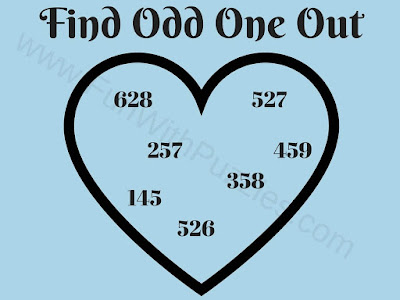## Tuesday, July 21, 2020

Here is one more Odd One Out Puzzle. In this challenging brain teaser, some numbers are given in heart shape. All the number except one number are related to each other. However, there is one number which does not relate to other numbers inside the heart. Can you find this Odd Number Out?Can you find Odd Number Out?

Answer to this "Challenging Brain Teaser", can be viewed by clicking on the button. Please do give your best try before looking at the answer.

1.Another Odd Number Out could be 459. All the other numbers give even number answers when all three numbers are multiplied together. But 459 gives an odd numbered answer.

1.If we multiply three digits in 459 we get 180 which is an even number :).

2.145, All the other numbers give even number answers when all three numbers are multiplied together. But 145 gives an odd numbered answer.

1.A comment doesn't cost a thing. Please drop a comment below to boost the author's morale.